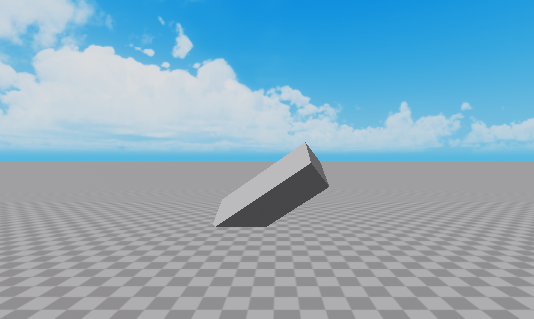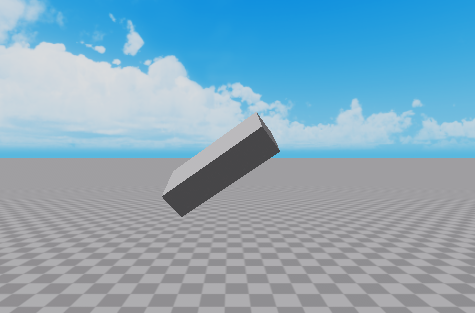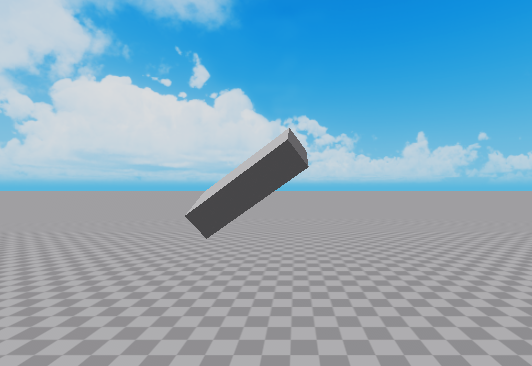# How To Use CFrame:VectorToWorldSpace() and CFrame:VectorToObjectSpace()

Hello!

I recently realized that the functions VectorToWorldSpace and VectorToObjectSpace are still the subject of confusion among many devs here on the forums. I remember when I used to have no clue how to use them, but after research and many hours of trial and error, I finally was able to understand them.
I couldn’t find too many other helpful articles on this, so I just decided to make my own.

https://developer.roblox.com/en-us/articles/CFrame-Math-Operations

This is how I think of them:

## VectorToWorldSpace :

``````myCFrame:VectorToWorldSpace(Vector3.new(0, 0, -1))
-- is the same exact thing as:
myCFrame.LookVector

myCFrame:VectorToWorldSpace(Vector3.new(0, 1, 0))
-- is the same exact thing as:
myCFrame.UpVector

myCFrame:VectorToWorldSpace(Vector3.new(-1, 0, 0))
-- is the same exact thing as:
-myCFrame.RightVector -- Notice the negative, (-RightVector is equal to LeftVector)
``````

If you didn’t use it, you have to write -LookVector to get the BackVector,
-RightVector to get the LeftVector, and so forth.

Using VectorToWorldSpace() gives you the flexibility to calculate many more directions than just Look, Right, Up, -Look, -Right, -Up, for example, if you wanted to find the direction 45 degrees between the LookVector and the RightVector.

To do this, now that we know that LookVector is

``````VectorToWorldSpace(Vector3.new(0, 0, -1))
``````

and RightVector is

``````VectorToWorldSpace(Vector3.new(1, 0, 0))
``````

and if you combined them into one Vector3:

``````VectorToWorldSpace(Vector3.new(1, 0, -1))
``````

What it would give you is this arrow directly between them:

Key:
A = Part.CFrame.LookVector
B = Part.CFrame.RightVector
C = Part.CFrame:VectorToWorldSpace(Vector3.new(1, 0, -1)) (45 degrees between Look and Right)

Although if you need to get one of these directional vectors with a magnitude of 1 (LookVector, -LookVector, RightVector, -RightVector, etc.) you should just those as opposed to VectorToWorldSpace, as it requires less computing power.

## VectorToObjectSpace :

If you read how to use VectorToWorldSpace, then all you need to know is that VectorToObjectSpace literally just does the opposite:

Thinking back to VectorToWorldSpace, now we know that MyCFrame.LookVector is the same exact thing as MyCFrame:VectorToWorldSpace(Vector3.new(0, 0, -1))

``````--print( MyPart.CFrame.LookVector )
0.707, -0, -0.707

--print( MyPart.CFrame:VectorToWorldSpace(Vector3.new(0, 0, -1)) )
0.707, -0, -0.707

``````

but now that we know where the part is facing in world space , (the 0.707, -0, -0.707 thing), if we put that into VectorToObjectSpace, watch what it prints:

``````--print( MyPart.CFrame:VectorToObjectSpace(Vector3.new(0.707, -0, -0.707)) )
0, 0, -1
``````

bam. The original LookVector (0, 0, -1)
This proves how VectorToObjectSpace() is the exact inverse of VectorToWorldSpace().

Check this out:

``````local directionThatMakesALookVector = Vector3.new(0, 0, -1)
local vectorToWorldSpaceResult = MyCFrame:VectorToWorldSpace(directionThatMakesALookVector)

--print(MyCFrame:VectorToObjectSpace(vectorToWorldSpaceResult))
0, 0, -1 -- equal to directionThatMakesALookVector, we got the original result!
``````

## Example of where VectorToObjectSpace can be used:You want to get the part here:(Directly one stud up)

(You would never actually want to do this for a case like this, you would just change the part’s Position property, but this is just to show what VectorToObjectSpace does)

You want to move it up using CFrame

if you did it like this,

``````part.CFrame = part.CFrame * CFrame.new(0, 1, 0) -- it will go 1 stud towards where the top is facing
``````

Since CFrame is dependent on the part’s rotation, it would go here:(it went up and left and towards the screen (towards the top face of the part), not directly one stud up)
To make it go up in world space, you could either do this:

``````part.CFrame = part.CFrame + Vector3.new(0, 1, 0)
-- or
part.Position = part.Position + Vector3.new(0, 1, 0)
-- or

local DistanceToTravelInWorldSpace = Vector3.new(0, 1, 0)

local StudsToTravelToGetThere = part.CFrame:VectorToObjectSpace(StudsToTravelToGetThere)

part.CFrame = part.CFrame * CFrame.new(StudsToTravelToGetThere)
``````

All three of these methods will make the part go exactly one stud up in world space.

I just thought I would give a lesson myself since I remember how confusing they were when I was first learning how to use them and I wanted to provide another resource for learning them.

If you have any questions or there is something you still are confused about, don’t be afraid to ask.

Hope you found this helpful everyone!

– Moonvane

60 Likes

I’m pretty sure it takes less time to write -LookVector but alright lol

Also, those vectors are properties of the orientation - they’re baked into the CFrame data. Calling `VectorToWorldSpace` is just extra computation if you don’t really need to use it for “more flexibility”. But I agree it helps to show what the function is doing.

For reference, there’s a good post here that explains it in more mathy-depth:

2 Likes

Didn’t include the computing thing, thanks for the heads up. It’s been updated

1 Like

Thank you very much. It cleared up some confusion for me.

1 Like

In this part, how does it know im talking about the Positional vectors? and not the directional vectors (XVector, ZVector, YVectors). Since a CFrame contains both positonal vectors, and directional vectors.

Is it possible to use ToObjectSpace to orientate a part based of an another part? I mean, can when I rotate a part, is there a way I can use this to rotate a part based off of The parts axis?